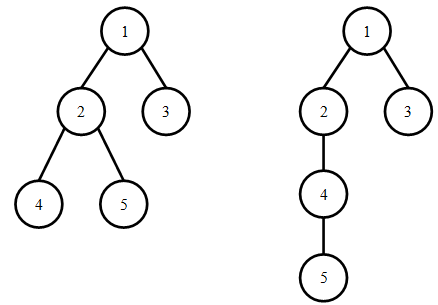시간 제한 메모리 제한 제출 정답 맞은 사람 정답 비율
1 초 512 MB 1 1 1 100.000%

문제

We know that a rooted tree can be traversed via depth-first search (DFS) and breadth-first search (BFS) to obtain the DFS and BFS orderings of their vertices. Two different trees can have the same DFS ordering, and at the same time their BFS orderings can also be the same. For example, the following two trees both have a DFS ordering of 1 2 4 5 3 and a BFS ordering of 1 2 3 4 5.Given a DFS and BFS ordering, we would like to know the average height of all rooted trees satisfying the condition. For example, if there are K different rooted trees simultaneously possessing the DFS and BFS orderings, and their heights are respectively h1h2, …, hK, then you are asked to output the value of (h1 + h2 + … + hK)/K.

입력

The first line contains a single positive integer n, representing the number of vertices.

The second line contains n positive integers (each between 1 and n, inclusive), representing the DFS ordering.

The third line contains n positive integers (each between 1 and n, inclusive), representing the BFS ordering.

The input guarantees that at least one tree satisfying the two orderings will exist.

출력

Output a single real number, representing the average height of the trees. The answer will be considered correct if the absolute error is at most 0.001.

If a rooted tree has only one vertex, then its height is 1. Otherwise, it's height is equal to 1 plus the maximum height across all of the subtrees rooted at each children.

2 ≤ n ≤ 200000

5
1 2 4 5 3
1 2 3 4 5

3.500